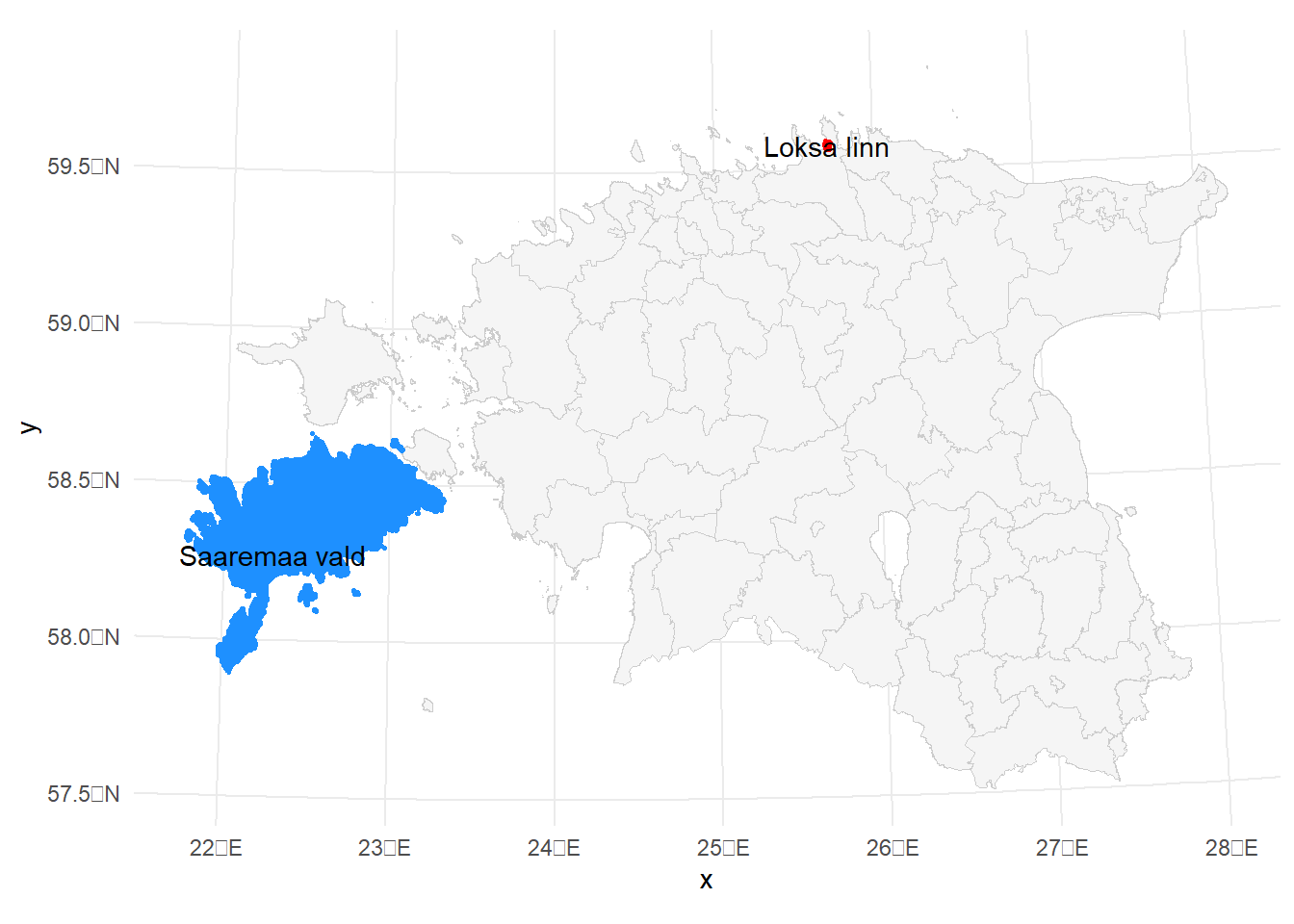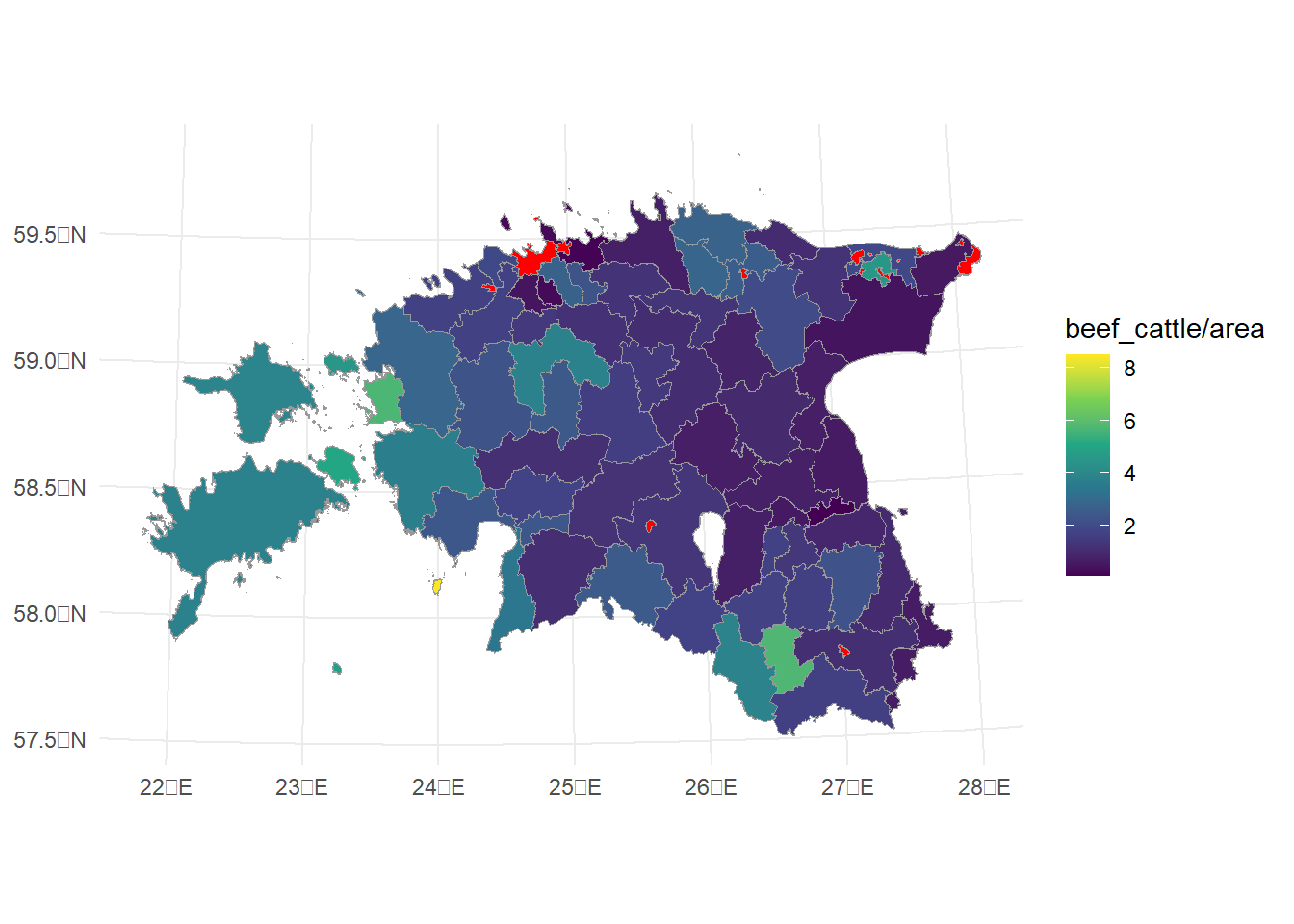Start libraries:

``````library(sf)
library(tidyverse)
library(rnaturalearth)
library(ggthemes)
library(knitr)
library(ggspatial)
library(tmap)
library(tmaptools)
library(lubridate)
library(raster)
library(rgeos)
library(ggmap)

options(scipen = 999)``````

# 1 Calculate area of polygon

In real life the spatial distribution of any phenomena is usually not regular. Irregular is also the distribution of administrative units. This means, if we want to compare different municipalities with each other, absolute values may not be the best option (at least we are then unable to describe the full picture). Next to the absolute values very often density is used. This means we present value per spatial unit (e.g. km2). Let us try to calculate areas of municipalities and then calculate in which municipality is the highest density of beef cattle:

``````# calculate area for each polygon:
municip\$area <- st_area(municip)

# Show areas of first 5 municipalities:
municip\$area[1:5]``````
``````## Units: [m^2]
##    11896170  207920558   73264580 2717872552 1032406180``````

We see that, polygon areas are calculated in same units as the reference system of geolayer (currently meters) and the variable type is Units. In current case it is reasonable to express it in square kilometers. We can convert units from square meter to square kilometer and store it as numeric:

``````municip <- municip %>%
mutate(area = as.numeric(area) / 1000000)

glimpse(municip)``````
``````## Rows: 79
## Columns: 7
## \$ ONIMI    <chr> "Ruhnu vald", "Muhu vald", "Viimsi vald", "Saaremaa vald", "H…
## \$ OKOOD    <chr> "0689", "0478", "0890", "0714", "0205", "0303", "0907", "0897…
## \$ MNIMI    <chr> "Saare maakond", "Saare maakond", "Harju maakond", "Saare maa…
## \$ MKOOD    <chr> "0074", "0074", "0037", "0074", "0039", "0068", "0056", "0084…
## \$ TYYP     <chr> "1", "1", "1", "1", "1", "1", "1", "4", "1", "4", "1", "4", "…
## \$ geometry <MULTIPOLYGON [m]> MULTIPOLYGON (((455191.3 64..., MULTIPOLYGON (((…
## \$ area     <dbl> 11.89617, 207.92056, 73.26458, 2717.87255, 1032.40618, 17.330…``````

Where is the biggest / smallest municipality (by area) of Estonia?

``````# filter biggest and smallest
municip_extreme <- municip %>%
filter(area == min(area) | area == max(area)) #"|" corresponds to 'OR'``````

Create the map (with `ggplot2`):

``````ggplot()+
theme_minimal()+
geom_sf(data = municip, fill="grey96", colour = "grey80", size = 0.25)+
geom_sf(data = municip_extreme, aes(fill = as.factor(area), colour = as.factor(area)), size=1)+
geom_sf_text(data = municip_extreme, aes(label=ONIMI))+
guides(fill=F, colour = F)+
scale_fill_manual(values =c("red", "dodgerblue"))+
scale_colour_manual(values =c("red", "dodgerblue"))``````
``````## Warning: `guides(<scale> = FALSE)` is deprecated. Please use `guides(<scale> =What is the area of biggest municipality? Smallest? What is average area of municipalities?

# 2 Spatial density

Let’s turn back to the geolayer of livestock in Estonia. First calculate the area of municipalities and convert it to square kilometers!

``# Calculate the area by your self!!!``

Hopefully it was easy! :)
In next step calculate the spatial density of beef cattle in municipalities:

``````# it can be done in several ways
# for example you can add calculation directly to the plotting syntax::
ggplot()+
theme_minimal()+
geom_sf(data = agrAnimal_2_sf_municip_aggr, aes(fill = beef_cattle / area), colour = "grey60", size = 0.25)+
scale_fill_viridis_c(na.value = "red")````````````# Or you can calculate the density as separate column and after that draw the map:
agrAnimal_2_sf_municip_aggr <- agrAnimal_2_sf_municip_aggr %>%
mutate(beef_dens = beef_cattle / area)

ggplot()+
theme_minimal()+
geom_sf(data = agrAnimal_2_sf_municip_aggr, aes(fill = beef_dens), colour = "grey60", size=0.25)+
scale_fill_viridis_c(na.value = "red")+
labs(fill = "animals\nper\nkm2")``````